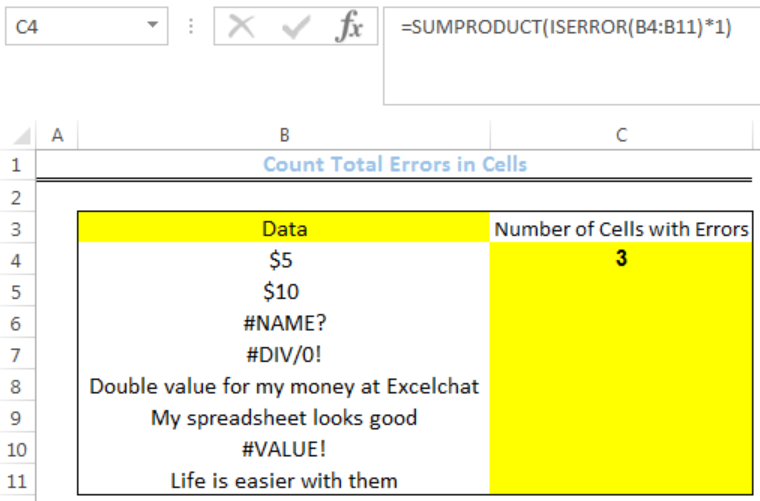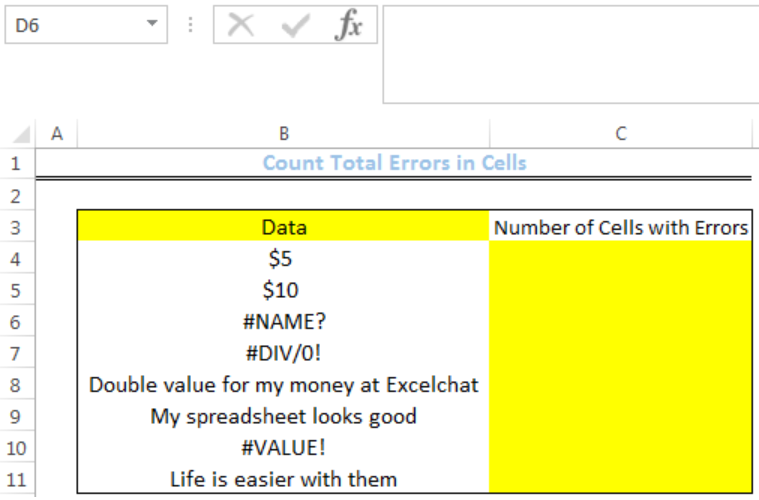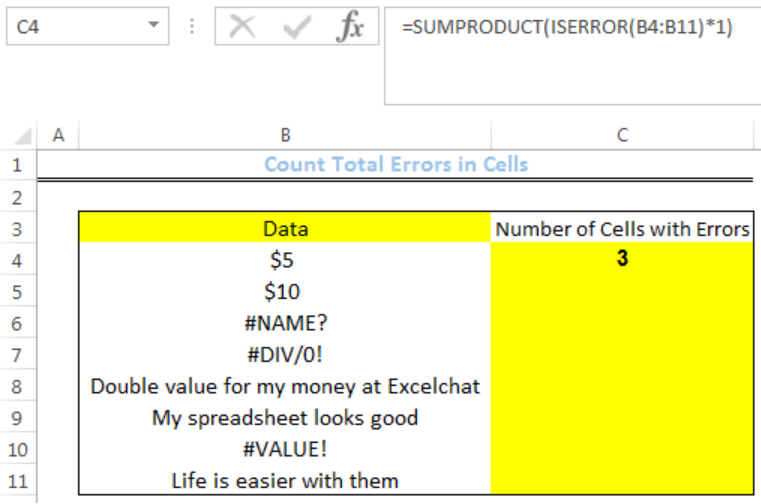Get instant live expert help with Excel or Google Sheets“My Excelchat expert helped me in less than 20 minutes, saving me what would have been 5 hours of work!”

#### Post your problem and you’ll get Expert help in seconds.

Your message must be at least 40 characters
Our professional Expert are available now. Your privacy is guaranteed.

# How to Count Cells that Contain ErrorsRead time: 29 minutes

We can Count Cells that Contain Errors in a range with a formula that combines the SUMPRODUCT and ISERROR functions. The steps below will walk through the process.Figure 1: How to Count Cells that Contain Errors

## General Formula:

`=SUMPRODUCT(ISERROR(range)*1)`

## Formula

`=SUMPRODUCT(ISERROR(B4:B11)*1)`

## Setting up the Data

• We will set up the data by inputting the DATA into Column B
• Cell C4 in Column C is where we want the formula to return the result for the number of cells with errorsFigure 2: Setting up the Data

## Count Cells that Contain Errors

• We will click on Cell C4
• We will insert the formula below into the cell
`=SUMPRODUCT(ISERROR(B4:B11)*1)`
• We will press the enter keyFigure 3: Count of Errors in Range B4:B11

## Explanation

The ISERROR function checks the range if they contain errors. The result is returned as an array of TRUE or FALSE.

`{FALSE;FALSE;TRUE;TRUE;FALSE;FALSE;TRUE,FALSE}`

TRUE represents the cells with errors and is converted to 1 so that the array looks like this:

`{0;0;1;1;0;0;1,0}`

The result of this array is then multiplied by 1 and the result is summed and returned as the result by the SUMPRODUCT Function.

## Note

We can use the SUM function instead of SUMPRODUCT function. However, we need to enter as an array formula using CTRL + SHIFT + ENTER. The formula is:

`=SUM(ISERROR(range)*1)`

ISERROR function counts all errors. If you use ISERR function, it will exclude #N/A

## Instant Connection to an Expert through our Excelchat Service

Most of the time, the problem you will need to solve will be more complex than a simple application of a formula or function. If you want to save hours of research and frustration, try our live Excelchat service! Our Excel Experts are available 24/7 to answer any Excel question you may have. We guarantee a connection within 30 seconds and a customized solution within 20 minutes.

### Did this post not answer your question? Get a solution from connecting with the expert.Another blog reader asked this question today on Excelchat:## Subscribe to Excelchat.coAnother blog reader asked this question today on Excelchat: